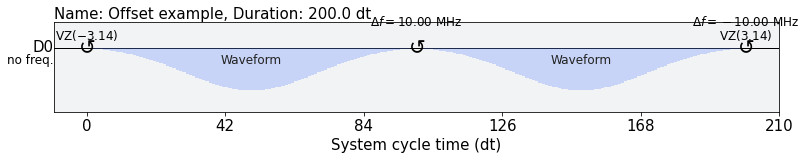# Pulse schedules

## Overview

Most quantum algorithms can be described with circuit operations alone. When you need more control over the low-level program implementation, you can use pulse gates. Pulse gates remove the constraint of executing circuits with basis gates only and let you override the default implementation of any basis gate.

Pulse gates let you map a logical circuit gate (for example, X) to a Qiskit Pulse program, called a ScheduleBlock. This mapping is referred to as a calibration. A high-fidelity calibration is one that faithfully implements the logical operation it is mapped from (for example, whether the X gate calibration drives $|0\rangle$ to $|1\rangle$).

A schedule specifies the exact time dynamics of the input signals across all input channels to the device. There are usually multiple channels per qubit, such as drive and measure. This interface is more powerful, and requires a deeper understanding of the underlying device physics.

It's important to note that pulse programs operate on physical qubits. A drive pulse on qubit $a$ does not enact the same logical operation on the state of qubit $b$. In other words, gate calibrations are not interchangeable across qubits. This is in contrast to the circuit level, where an X gate is defined independently of its qubit operand.

Note: Not all providers support pulse gates.

 :
from qiskit import QuantumCircuit

circ = QuantumCircuit(2, 2)
circ.h(0)
circ.cx(0, 1)
circ.measure(0, 0)
circ.measure(1, 1)

circ.draw('mpl')

Output: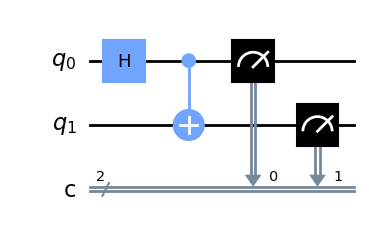Define a calibration for the Hadamard gate on qubit 0.

In practice, the pulse shape and its parameters would be optimized through a series of calibration experiments. For this demonstration, the Hadamard will be a Gaussian pulse. You play the pulse on the drive channel of qubit 0.

For more information on calibrations, see the Qiskit Experiments tutorial. (opens in a new tab)

 :
from qiskit import pulse
from qiskit.pulse.library import Gaussian
from qiskit.providers.fake_provider import FakeValencia

backend = FakeValencia()

pulse.play(Gaussian(duration=128, amp=0.1, sigma=16), pulse.drive_channel(0))

Let's draw the new schedule to see what we've built.

 :
h_q0.draw()

Output: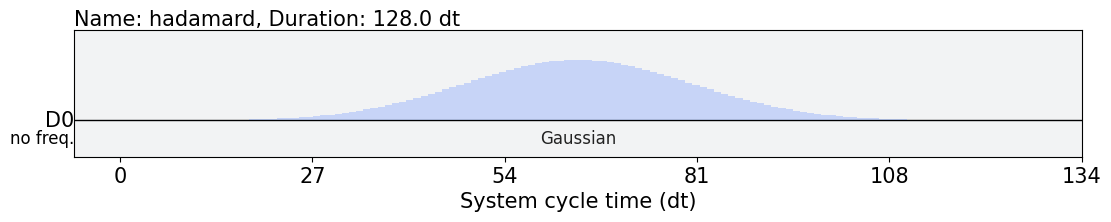All that remains is to complete the registration. The circuit method add_calibration needs information about the gate and a reference to the schedule to complete the mapping:

QuantumCircuit.add_calibration(gate, qubits, schedule, parameters)

The gate can be either a circuit.Gate object or the name of the gate. Usually, you'll need a different schedule for each unique set of qubits and parameters. Since the Hadamard gate doesn't have any parameters, there is no need to supply any.

 :
circ.add_calibration('h', , h_q0)

Lastly, note that the transpiler will respect your calibrations. Use it as you normally would (our example is too simple for the transpiler to optimize, so the output is the same).

 :
from qiskit import transpile
from qiskit.providers.fake_provider import FakeHanoi

backend = FakeHanoi()

circ = transpile(circ, backend)

print(backend.configuration().basis_gates)
circ.draw('mpl', idle_wires=False)

Output:

['id', 'rz', 'sx', 'x', 'cx', 'reset']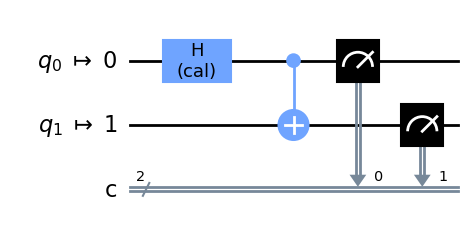Notice that h is not a basis gate for the mock backend FakeHanoi. Since you added a calibration for it, the transpiler will treat the gate as a basis gate, but only on the qubits for which it was defined. A Hadamard applied to a different qubit would be unrolled to the basis gates.

### Custom gates

This demonstrates the same process for nonstandard, completely custom gates, including a gate with parameters.

 :
from qiskit import QuantumCircuit
from qiskit.circuit import Gate

circ = QuantumCircuit(1, 1)
custom_gate = Gate('my_custom_gate', 1, [3.14, 1])
# 3.14 is an arbitrary parameter for demonstration
circ.append(custom_gate, )
circ.measure(0, 0)

circ.draw('mpl')

Output: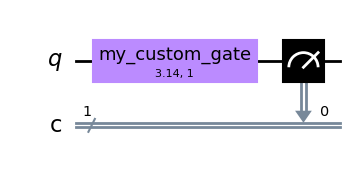:
with pulse.build(backend, name='custom') as my_schedule:
pulse.play(Gaussian(duration=64, amp=0.2, sigma=8), pulse.drive_channel(0))

# Alternatively: circ.add_calibration(custom_gate, , my_schedule)

If you use the Gate instance variable custom_gate to add the calibration, the parameters are derived from that instance. Remember that the order of parameters is significant.

 :
circ = transpile(circ, backend)
circ.draw('mpl', idle_wires=False)

Output: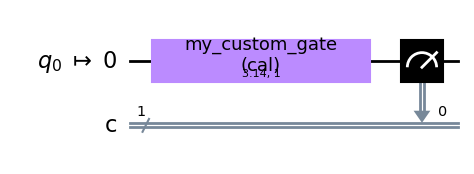Normally, if you tried to transpile circ, you would get an error. There was no functional definition provided for "my_custom_gate", so the transpiler can't unroll it to the basis gate set of the target device. You can show this by trying to add "my_custom_gate" to another qubit that hasn't been calibrated.

 :
circ = QuantumCircuit(2, 2)
circ.append(custom_gate, )

from qiskit import QiskitError
try:
circ = transpile(circ, backend)
except QiskitError as e:
print(e)

Output:

"Cannot unroll the circuit to the given basis, ['id', 'rz', 'sx', 'x', 'cx', 'reset']. Instruction my_custom_gate not found in equivalence library and no rule found to expand."


To link a custom gate to your circuits, you can also add to Target and transpile. A pass manager pass implicitly extracts calibration data from the target and calls add_calibration. This is convenient if you need to attach a calibration to multiple circuits or manage multiple calibrations.

[ ] :
from qiskit.providers.fake_provider import FakePerth
from qiskit.circuit import QuantumCircuit, Gate
from qiskit.pulse import builder, DriveChannel
from qiskit.compiler import transpile
from qiskit.transpiler import InstructionProperties

backend = FakePerth()

custom_gate = Gate("my_gate", 1, [])
qc = QuantumCircuit(1, 1)
qc.append(custom_gate, )
qc.measure(0, 0)

with builder.build() as custom_sched_q0:
builder.play([0.1] * 160, DriveChannel(0))

custom_gate,
{(0,): InstructionProperties(calibration=custom_sched_q0)},
)

qc = transpile(qc, backend)

## Build pulse schedules

Pulse gates define a low-level, exact representation for a circuit gate. A single operation can be implemented with a pulse program, which is comprised of multiple low-level instructions. Regardless of how the program is used, the syntax for building the program is the same.

Important: For IBM devices, pulse programs are used as subroutines to describe gates. IBM devices do not accept full programs in this format.

A pulse program, which is called a ScheduleBlock, describes instruction sequences for the control electronics. Use the Pulse Builder to build a ScheduleBlock, then initialize a schedule:

[ ] :
from qiskit import pulse

with pulse.build(name='my_example') as my_program:
pass

my_program

Output:

ScheduleBlock(, name="my_example", transform=AlignLeft())


You can see that there are no instructions yet. The next section explains each of the instructions you might add to a schedule, and the last section will describe various alignment contexts, which determine how instructions are placed in time relative to one another.

### ScheduleBlock Instructions

Each instruction type has its own set of operands. As you can see above, they each include at least one Channel to specify where the instruction will be applied.

Channels are labels for signal lines from the control hardware to the quantum chip.

• A DriveChannel is typically used for driving single-qubit rotations.
• A ControlChannel is typically used for multi-qubit gates or additional drive lines for tunable qubits.
• A MeasureChannel is specific to transmitting pulses that stimulate readout.
• An AcquireChannel is used to trigger digitizers which collect readout signals.

DriveChannels, ControlChannels, and MeasureChannels are all PulseChannels; this means that they support transmitting pulses, whereas the AcquireChannel is a receive channel only and cannot play waveforms.

In the following examples, you can create one DriveChannel instance for each Instruction that accepts a PulseChannel. Channels take one integer index argument. Except for ControlChannels, the index maps trivially to the qubit label.

[ ] :
from qiskit.pulse import DriveChannel

channel = DriveChannel(0)

The pulse ScheduleBlock is independent of the backend it runs on. However, you can build your program in a context that is aware of the target backend by supplying it to pulse.build. When possible you should supply a backend. By using the channel accessors pulse.<type>_channel(<idx>) you ensure you are only using available device resources.

[ ] :
from qiskit.providers.fake_provider import FakeValencia

backend = FakeValencia()

with pulse.build(backend=backend, name='backend_aware') as backend_aware_program:
channel = pulse.drive_channel(0)
print(pulse.num_qubits())
# Raises an error as backend only has 5 qubits
#pulse.drive_channel(100)

Output:

5


### delay

One of the simplest instructions is delay. This is a blocking instruction that tells the control electronics to output no signal on the given channel for the duration specified. It is useful for controlling the timing of other instructions.

The duration here and elsewhere is in terms of the backend's cycle time (1 / sample rate), dt. It must take an integer value.

To add a delay instruction, pass a duration and a channel, where channel can be any kind of channel, including AcquireChannel. Use pulse.build to begin a Pulse Builder context. This automatically schedules the delay into the schedule delay_5dt.

[ ] :
with pulse.build(backend) as delay_5dt:
pulse.delay(5, channel)

Any instruction added after this delay on the same channel will execute five timesteps later than it would have without this delay.

### play

The play instruction is responsible for executing pulses. It's straightforward to add a play instruction:

with pulse.build() as sched:
pulse.play(pulse, channel)


Let's clarify what the pulse argument is and explore a few different ways to build one.

#### Pulses

A Pulse specifies an arbitrary pulse envelope. The modulation frequency and phase of the output waveform are controlled by the set_frequency and shift_phase instructions.

There are many methods available for building pulses, such as those available in the Qiskit Pulse library. Take for example a simple Gaussian pulse -- a pulse with its envelope described by a sampled Gaussian function. We arbitrarily choose an amplitude of 1, standard deviation $\sigma$ of 10, and 128 sample points.

Note: The amplitude norm is arbitrarily limited to 1.0. Each backend system may also impose further constraints. For instance, a minimum pulse size of 64. Any additional constraints are provided through Target.

[ ] :
from qiskit.pulse import library

amp = 1
sigma = 10
num_samples = 128
##### Parametric pulses

You can build a Gaussian pulse by using the Gaussian parametric pulse. A parametric pulse sends the name of the function and its parameters to the backend, rather than every individual sample. Using parametric pulses makes the jobs much smaller to send. IBM Quantum backends limit the maximum job size that they accept, so parametric pulses might allow you to run larger programs.

Other parametric pulses in the library include GaussianSquare, Drag, and Constant. See the full list in the API reference.

Note: The backend is responsible for deciding how to sample the parametric pulses. It is possible to draw parametric pulses, but the samples displayed are not guaranteed to be the same as those executed on the backend.

[ ] :
gaus = pulse.library.Gaussian(num_samples, amp, sigma,
name="Parametric Gaus")
gaus.draw()

Output: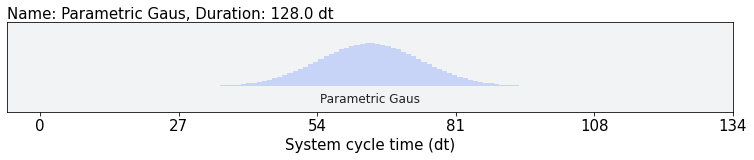##### Pulse waveforms described by samples

A Waveform is a pulse signal specified as an array of time-ordered complex amplitudes, or samples. Each sample is played for one cycle, a timestep dt, determined by the backend. You must know the value of dt to determine a program's real-time dynamics. The (zero-indexed) $i^{th}$ sample plays from time i*dt up to (i + 1)*dt, modulated by the qubit frequency.

[ ] :
import numpy as np

times = np.arange(num_samples)
gaussian_samples = np.exp(-1/2 *((times - num_samples / 2) ** 2 / sigma**2))

gaus = library.Waveform(gaussian_samples, name="WF Gaus")
gaus.draw()

Output: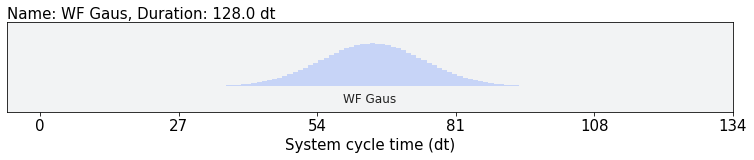Regardless of which method you use to specify your pulse, play is added to your schedule the same way:

[ ] :
with pulse.build() as schedule:
pulse.play(gaus, channel)
schedule.draw()

Output: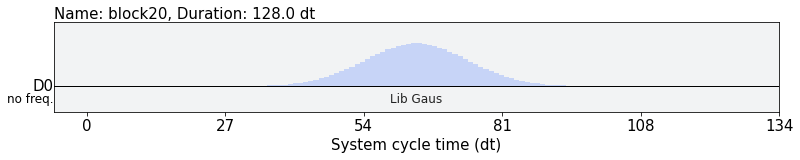You may also supply a complex list or array directly to play.

[ ] :
with pulse.build() as schedule:
pulse.play([0.001*i for i in range(160)], channel)
schedule.draw()

Output: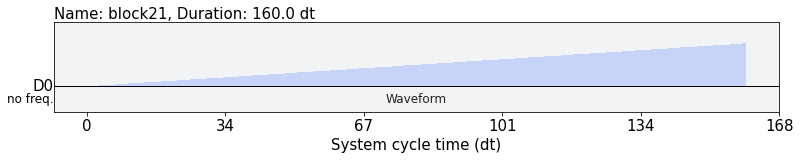The play instruction gets its duration from its Pulse: the duration of a parametrized pulse is an explicit argument, and the duration of a Waveform is the number of input samples.

### set_frequency

As explained previously, the output pulse waveform envelope is also modulated by a frequency and phase. Each channel has a default frequency listed in the backend.defaults.

A channel's frequency can be updated at any time within a ScheduleBlock by the set_frequency instruction. It takes a float frequency and a PulseChannel channel as input. All pulses on a channel following a set_frequency instruction are modulated by the given frequency until another set_frequency instruction is encountered or until the program ends.

The instruction has an implicit duration of 0.

Note: The frequencies that can be requested are limited by the total bandwidth and the instantaneous bandwidth of each hardware channel. In the future, these will be reported by the backend.

[ ] :
with pulse.build(backend) as schedule:
pulse.set_frequency(4.5e9, channel)

### shift_frequency

The shift_frequency instruction shifts the frequency of a pulse channel.

[ ] :
d0 = pulse.DriveChannel(0)

with pulse.build() as pulse_prog:
pulse.shift_frequency(1e9, d0)
Note

The shift_frequency and set_frequency instructions change the frequency of following pulses and also change the channel's frame of reference. Because a qubit oscillates at its transition frequency, the controller needs to sync with its oscillation; otherwise, an unwanted Z drive is continuously applied. Usually, because the frame is matched with the drive's frequency, and drive matches with the transition's frequency, the Z drive is eliminated when the qubit frequency is calibrated properly. When you apply the shift_frequency instruction, it changes the drive frequency and impacts the frame. In other words, it accumulates the phase (Z) as a function of shifted frequency and duration of the program. Specifically, when you shift the frequency by df and spend dt on that frame, the qubit may experience a phase rotation of df * dt. The programmer needs to take this into account to control their qubits precisely.

Note also that these instructions are localized in the pulse gate in IBM devices. This means that accumulated phase and frequency shifts are not carried over. Each pulse gate always starts from the hardware default setting. This behavior is backend-dependent.

### set_phase

The set_phase instruction sets the phase of a pulse channel.

[ ] :
d0 = pulse.DriveChannel(0)

with pulse.build() as pulse_prog:
pulse.set_phase(math.pi, d0)

### shift_phase

The shift_phase instruction will increase the phase of the frequency modulation by phase. Like set_frequency, this phase shift will affect all following instructions on the same channel until the program ends. To undo the affect of a shift_phase, the negative phase can be passed to a new instruction.

Like set_frequency, the instruction has an implicit duration of 0.

[ ] :
with pulse.build(backend) as schedule:
pulse.shift_phase(np.pi, channel)

### acquire

The acquire instruction triggers data acquisition for readout. It takes a duration, an AcquireChannel, which maps to the qubit being measured, and a MemorySlot or a RegisterSlot. The MemorySlot is classical memory where the readout result will be stored. The RegisterSlot maps to a register in the control electronics that stores the readout result for fast feedback.

The acquire instruction can also take custom Discriminators and Kernels as keyword arguments. The Kernel subroutine integrates a time series of measurement responses and generates an IQ data point, which will be classified into a quantum state by the discriminator. This indicates that if you use a custom measurement stimulus, as in a measurement pulse, you might need to update the kernel setting to not deteriorate the measurement SNR.

[ ] :
from qiskit.pulse import Acquire, AcquireChannel, MemorySlot

with pulse.build(backend) as schedule:
pulse.acquire(1200, pulse.acquire_channel(0), MemorySlot(0))

After adding ScheduleBlock instructions, you need to understand how to control when they're played.

## Pulse Builder

Below are the most important Pulse Builder features for learning how to build schedules. This is not an exhaustive list. For more details about using the Pulse Builder, refer to the Pulse API reference.

### Alignment contexts

The builder has alignment contexts that influence how a schedule is built. Contexts can also be nested. Try them out, and use .draw() to see how the pulses are aligned.

Regardless of the alignment context, the duration of the resulting schedule is as short as it can be while including every instruction and following the alignment rules. This still allows some degrees of freedom for scheduling instructions off the "longest path". The examples below illustrate this.

### align_left

The builder has alignment contexts that influence how a schedule is built. The default is align_left.

[ ] :
with pulse.build(backend, name='Left align example') as program:
with pulse.align_left():
gaussian_pulse = library.Gaussian(100, 0.5, 20)
pulse.play(gaussian_pulse, pulse.drive_channel(0))
pulse.play(gaussian_pulse, pulse.drive_channel(1))
pulse.play(gaussian_pulse, pulse.drive_channel(1))

program.draw()

Output: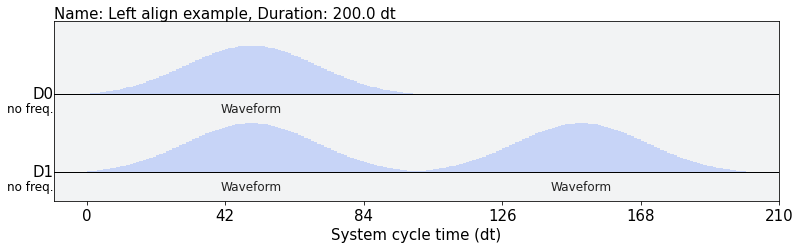Notice how there is no scheduling freedom for the pulses on D1. The second waveform begins immediately after the first. The pulse on D0 can start at any time between t=0 and t=100 without changing the duration of the overall schedule. The align_left context sets the start time of this pulse to t=0. You can think of this like left-justification of a text document.

### align_right

align_right does the opposite of align_left. It chooses t=100 in the above example to begin the Gaussian pulse on D0. Left and right are also sometimes called "as soon as possible" and "as late as possible" scheduling, respectively.

[ ] :
with pulse.build(backend, name='Right align example') as program:
with pulse.align_right():
gaussian_pulse = library.Gaussian(100, 0.5, 20)
pulse.play(gaussian_pulse, pulse.drive_channel(0))
pulse.play(gaussian_pulse, pulse.drive_channel(1))
pulse.play(gaussian_pulse, pulse.drive_channel(1))

program.draw()

Output: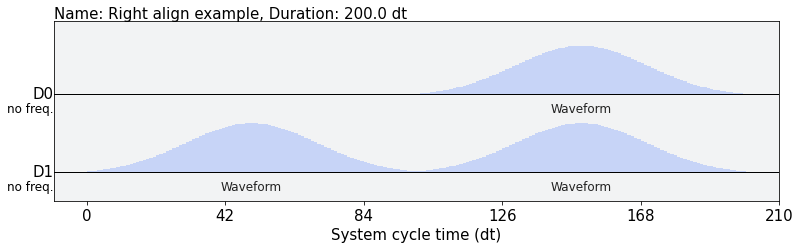### align_equispaced(duration)

If the duration of a particular block is known, you can also use align_equispaced to insert equal duration delays between each instruction.

[ ] :
with pulse.build(backend, name='example') as program:
gaussian_pulse = library.Gaussian(100, 0.5, 20)
with pulse.align_equispaced(2*gaussian_pulse.duration):
pulse.play(gaussian_pulse, pulse.drive_channel(0))
pulse.play(gaussian_pulse, pulse.drive_channel(1))
pulse.play(gaussian_pulse, pulse.drive_channel(1))

program.draw()

Output: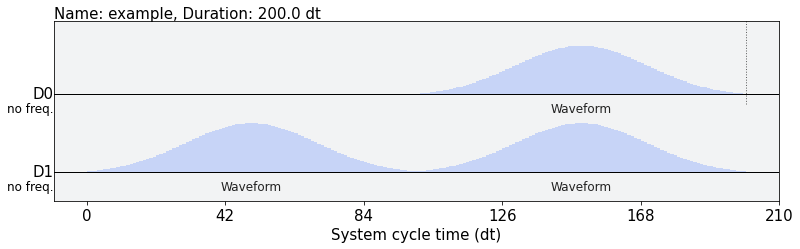### align_sequential

This alignment context does not schedule instructions in parallel. Each instruction will begin at the end of the previously added instruction.

[ ] :
with pulse.build(backend, name='example') as program:
with pulse.align_sequential():
gaussian_pulse = library.Gaussian(100, 0.5, 20)
pulse.play(gaussian_pulse, pulse.drive_channel(0))
pulse.play(gaussian_pulse, pulse.drive_channel(1))
pulse.play(gaussian_pulse, pulse.drive_channel(1))

program.draw()

Output: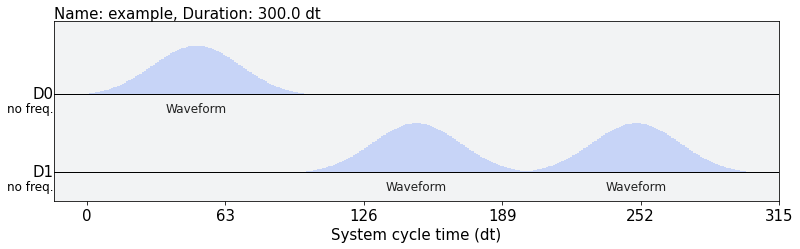### Phase and frequency offsets

The builder can help temporarily offset the frequency or phase of pulses on a channel.

[ ] :
with pulse.build(backend, name='Offset example') as program:
with pulse.phase_offset(3.14, pulse.drive_channel(0)):
pulse.play(gaussian_pulse, pulse.drive_channel(0))
with pulse.frequency_offset(10e6, pulse.drive_channel(0)):
pulse.play(gaussian_pulse, pulse.drive_channel(0))

program.draw()

Output: